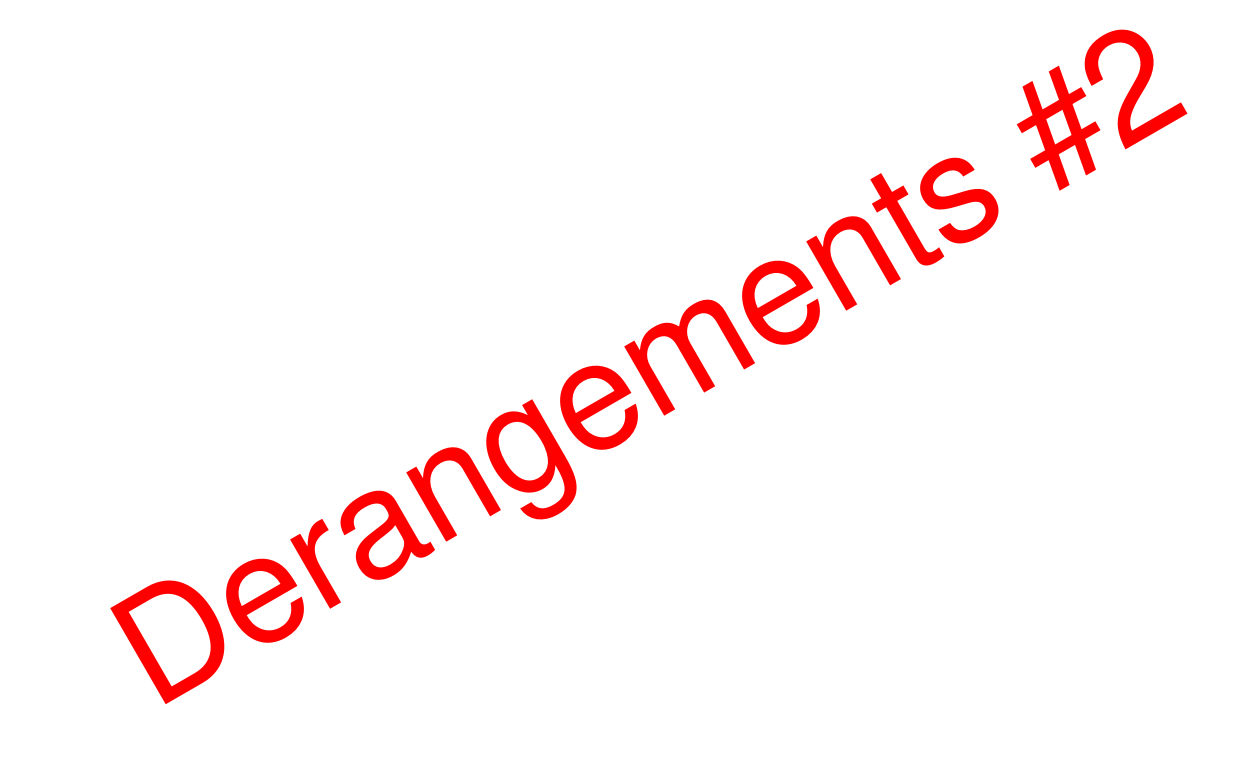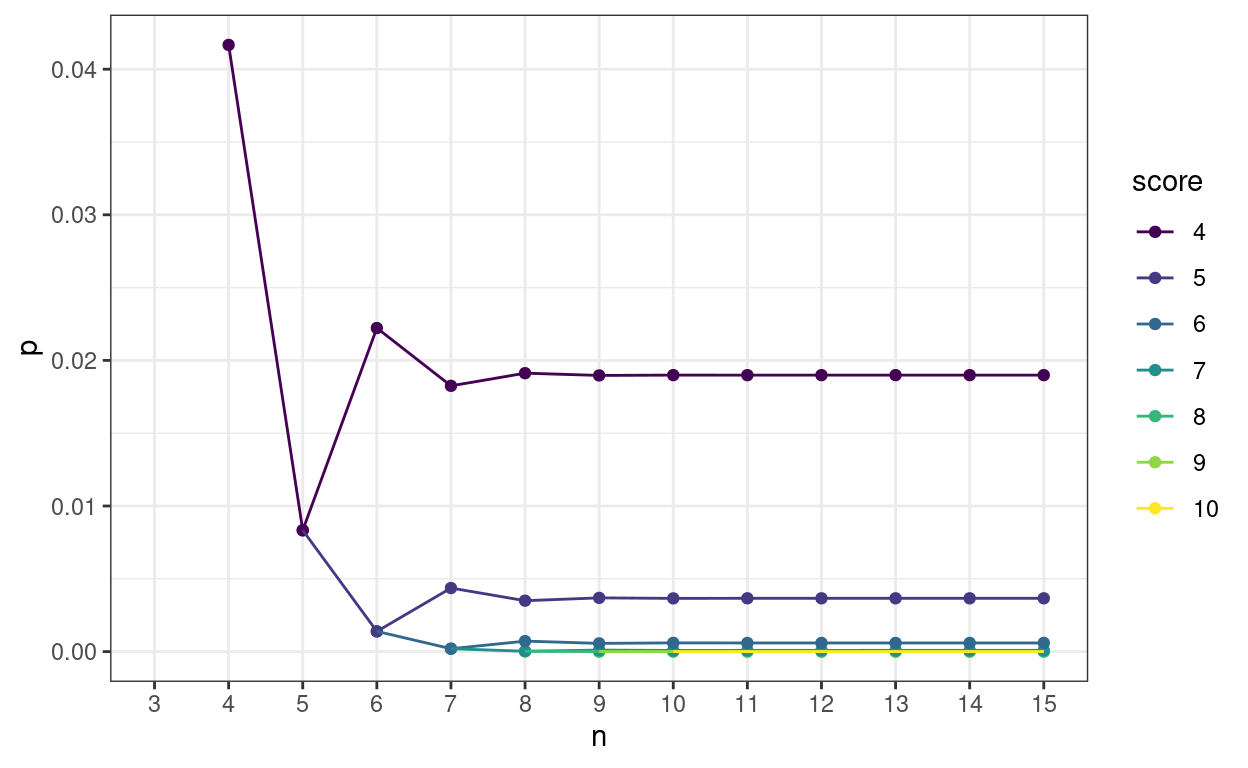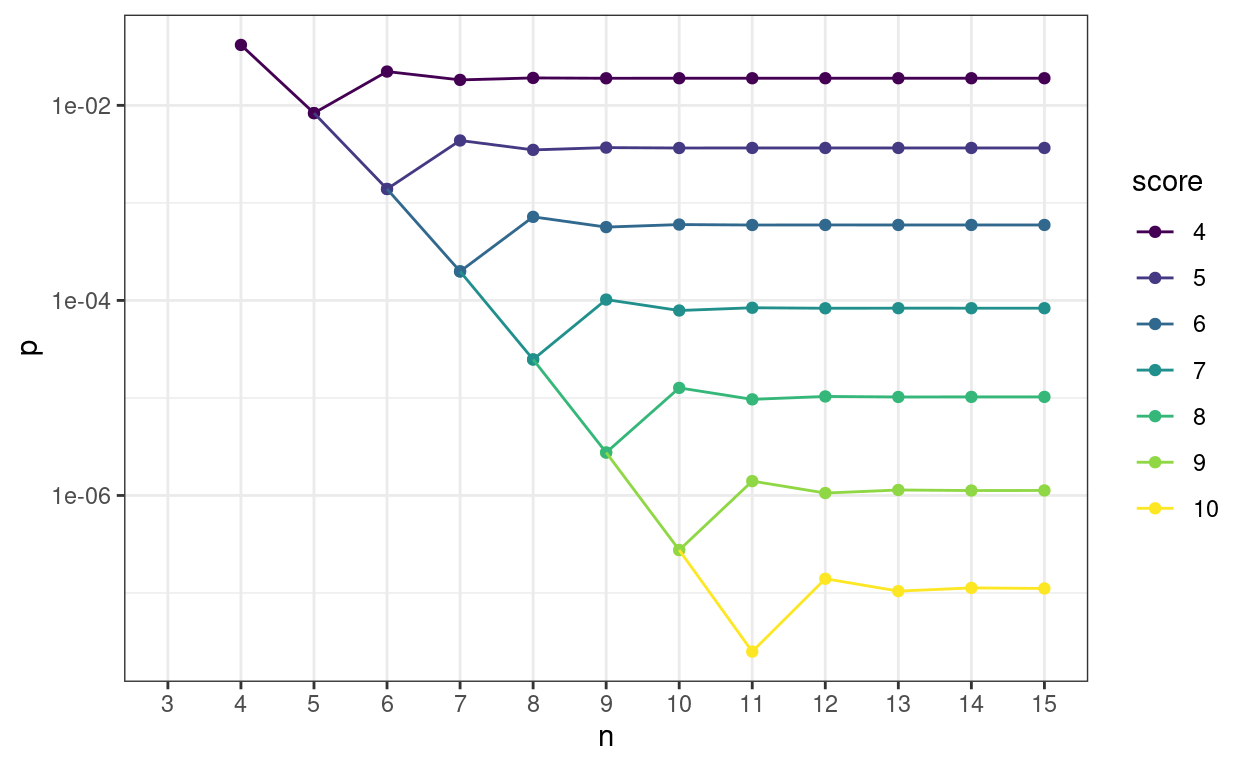# Derangements #2

Follows on from ‘Scores from matching things’

Chris Evans https://www.psyctc.org/R_blog/ (PSYCTC.org)https://www.psyctc.org/psyctc/
2022-07-23
Show code
``````as_tibble(list(x = 1,
y = 1)) -> tibDat

ggplot(data = tibDat,
aes(x = x, y = y)) +
geom_text(label = "Derangements #2",
size = 20,
colour = "red",
angle = 30,
lineheight = 1) +
xlab("") +
ylab("") +
theme_bw() +
theme(panel.grid.major = element_blank(),
panel.grid.minor = element_blank(),
panel.border = element_blank(),
panel.background = element_blank(),
axis.line = element_blank(),
axis.text = element_blank(),
axis.ticks = element_blank()) ``````In my last post here, Scores from matching things I gave the background to the probabilities of achieving certain scores on matching tasks by chance alone to help explain the perhaps counter-intuitive finding that matching four or more things correctly is unlikely by chance alone at p < .05 regardless of the number of objects to be matched.

This just adds a bit more to that, mostly as plots and complements both that Rblog post and an “ordinary” blog post, Sometimes n=4 is enough.

What I wanted was to show how rapidly the probabilities of achieving any particular score stabilise to an asymptotic value as n increases. Here we are for n from 4 to 15 and scores from 4 to 10.

Show code
``````### create some functions (as in previous post)
all.derangements <- function(n){
cumprob <- prob <- number <- term <- score <- rev(0:n)
for (m in 1:n) {
i <- m+1
s <- n-m
term[i] <- ((-1)^(m))/(factorial(m))
}
term <- 1
for (i in 0:n) {
s <- i+1
prob[s] <- (sum(term[1:s]))/factorial(n-i)
}
number <- factorial(n)*prob
for (s in 0:n) {
m <- n-s
i <- m+1
cumprob[i] <- sum(prob[1:i])
}
tmp <- cbind(n, score,number,prob,cumprob)
tmp
}

p.derange.score <- function(score,n){
if (score > n) stop("Score cannot be greater than n")
if (score == (n-1)) stop ("Score cannot be n-1")
cumprob <- prob <- term <- rev(0:n)
for (m in 1:n) {
i <- m+1
s <- n-m
term[i] <- ((-1)^(m))/(factorial(m))
}
term <- 1
for (i in 0:n) {
s <- i+1
prob[s] <- (sum(term[1:s]))/factorial(n-i)
}
for (s in 0:n) {
m <- n-s
i <- m+1
cumprob[i] <- sum(prob[1:i])
}
cumprob[n+1-score]
}

### now let's go a bit further
### get all the possible scores for n from 4 to 30
lapply(4:30, FUN = all.derangements) -> tmpList
### I always forget this nice little bit of base R and I'm a bit surprised that there doesn't seem to be a nice tidyverse alternative
do.call(rbind.data.frame, tmpList) %>%
as_tibble() -> tmpTib
### this was just to produce some tables for my blog post at https://www.psyctc.org/psyctc/2022/07/23/sometimes-n4-is-enough/
# tmpTib %>%
#   write_csv(file = "derangements.csv")

### ditto
# 1:14 %>%
#   as_tibble() %>%
#   rename(n = value) %>%
#   mutate(PossibleWays = factorial(n),
#          PossibleWays = prettyNum(PossibleWays, big.mark = ",")) %>%
#   write_csv(file = "numbers.csv")

### but Vectorizing the function seemed cleaner so ...
Vectorize(FUN = all.derangements) -> All.derangements
All.derangements(4:14) -> tmpList
### back to the do.call() just for the tables
# do.call(rbind, tmpList) %>%
#   as_tibble() %>%
#   filter(score == 4) %>%
#   select(n, number) %>%
#   mutate(number = prettyNum(number, big.mark = ",")) %>%
#   write_csv("correct.csv")

# do.call(rbind, tmpList) %>%
#   as_tibble() %>%
#   filter(score == 4) %>%
#   mutate(totalPerms = factorial(n)) %>%
#   select(-prob) %>%
#   select(n, totalPerms, everything()) %>%
#   write_csv("final.csv")

### OK, now for this Rblog post!
All.derangements(4:15) -> tmpList
do.call(rbind, tmpList) %>%
as_tibble() %>%
filter(score > 3 & score < 11) %>%
mutate(score = ordered(score,
levels = 4:10)) -> tmpTib

ggplot(data = tmpTib,
aes(x = n, y = cumprob, colour = score, group = score)) +
geom_point() +
geom_line() +
scale_x_continuous(breaks = 3:15,
minor_breaks = 3:15,
limits = c(3, 15)) +
ylab("p") +
theme_bw() ``````Here’s the same on a log10 y axis to separate the p values for the higher scores.

Show code
``````ggplot(data = tmpTib,
aes(x = n, y = cumprob, colour = score, group = score)) +
geom_point() +
geom_line() +
scale_x_continuous(breaks = 3:15,
minor_breaks = 3:15,
limits = c(3, 15)) +
scale_y_continuous(trans = "log10") +
ylab("p") +
theme_bw() ``````This next table shows how rapidly p values of real interest stabilise. The table is ordered by number of objects (n) within score. The column p is the probability of getting that score or better by chance alone, diffProb is the absolute change in that p value from the one for the previous n, diffPerc is the difference as a percentage of the previous p value. diffProbLT001 flags when the change in absolute p vaue is below .001 at which point I think in my realm any further precision is spurious. However, diffLT1pct flags when the change in p value is below 1% of the previous p value just in case someone wants that sort precise convergence.

Show code
``````All.derangements(4:20) -> tmpList
do.call(rbind, tmpList) %>%
as_tibble() %>%
filter(score > 3 & score < 11)  -> tmpTib

### just working out how stable the p values get how soon
tmpTib %>%
arrange(score) %>%
group_by(score) %>%
mutate(diffProb = abs(cumprob - lag(cumprob)),
diffProbLT001 = if_else(diffProb < .001, "Y", "N"),
diffPerc = 100 * diffProb /lag(cumprob),
diffLT1pct = if_else(diffPerc < 1, "Y", "N")) %>%
ungroup() %>%
select(-c(number, prob)) %>%
rename(p = cumprob) %>%
select(score, everything()) %>%
as_hux() %>%
set_position("left") %>% # left align the whole table
set_bold(row = everywhere, col = everywhere) %>% # everything into bold
set_align(everywhere, everywhere, "center") %>% # everything centred
set_align(everywhere, 1:2, "right") %>% # but now right justify the first two columns
map_text_color(by_values("Y" = "green")) %>% # colour matches by text recognition
map_text_color(by_values("N" = "red"))``````
scorenpdiffProbdiffProbLT001diffPercdiffLT1pct
440.0417
450.008330.0333N80N
460.02220.0139N167N
470.01830.00397N17.9N
480.01910.000868Y4.76N
490.0190.000154Y0.807Y
4100.0192.31e-05Y0.122Y
4110.0193.01e-06Y0.0158Y
4120.0193.44e-07Y0.00181Y
4130.0193.53e-08Y0.000186Y
4140.0193.28e-09Y1.73e-05Y
4150.0192.78e-10Y1.47e-06Y
4160.0192.17e-11Y1.15e-07Y
4170.0191.57e-12Y8.29e-09Y
4180.0191.06e-13Y5.59e-10Y
4190.0196.71e-15Y3.53e-11Y
4200.0193.99e-16Y2.1e-12Y
550.00833
560.001390.00694N83.3N
570.004370.00298N214N
580.00350.000868Y19.9N
590.003690.000193Y5.52N
5100.003663.47e-05Y0.941Y
5110.003665.26e-06Y0.144Y
5120.003666.89e-07Y0.0188Y
5130.003667.95e-08Y0.00217Y
5140.003668.2e-09Y0.000224Y
5150.003667.65e-10Y2.09e-05Y
5160.003666.52e-11Y1.78e-06Y
5170.003665.12e-12Y1.4e-07Y
5180.003663.72e-13Y1.02e-08Y
5190.003662.52e-14Y6.87e-10Y
5200.003661.59e-15Y4.35e-11Y
660.00139
670.0001980.00119N85.7N
680.0007190.000521Y262N
690.0005650.000154Y21.5N
6100.00063.47e-05Y6.15N
6110.0005936.31e-06Y1.05N
6120.0005949.65e-07Y0.163Y
6130.0005941.27e-07Y0.0214Y
6140.0005941.48e-08Y0.00248Y
6150.0005941.53e-09Y0.000258Y
6160.0005941.44e-10Y2.42e-05Y
6170.0005941.23e-11Y2.07e-06Y
6180.0005949.67e-13Y1.63e-07Y
6190.0005947.04e-14Y1.19e-08Y
6200.0005944.78e-15Y8.04e-10Y
770.000198
782.48e-050.000174Y87.5N
790.0001027.72e-05Y311N
7107.88e-052.31e-05Y22.7N
7118.41e-055.26e-06Y6.68N
7128.31e-059.65e-07Y1.15N
7138.33e-051.48e-07Y0.179Y
7148.32e-051.97e-08Y0.0236Y
7158.32e-052.3e-09Y0.00276Y
7168.32e-052.39e-10Y0.000287Y
7178.32e-052.25e-11Y2.7e-05Y
7188.32e-051.93e-12Y2.32e-06Y
7198.32e-051.53e-13Y1.83e-07Y
7208.32e-051.12e-14Y1.34e-08Y
882.48e-05
892.76e-062.2e-05Y88.9N
8101.27e-059.92e-06Y360N
8119.67e-063.01e-06Y23.7N
8121.04e-056.89e-07Y7.12N
8131.02e-051.27e-07Y1.23N
8141.03e-051.97e-08Y0.192Y
8151.02e-052.62e-09Y0.0256Y
8161.02e-053.08e-10Y0.003Y
8171.02e-053.22e-11Y0.000314Y
8181.02e-053.04e-12Y2.96e-05Y
8191.02e-052.62e-13Y2.55e-06Y
8201.02e-052.07e-14Y2.02e-07Y
992.76e-06
9102.76e-072.48e-06Y90N
9111.4e-061.13e-06Y409N
9121.06e-063.44e-07Y24.6N
9131.14e-067.95e-08Y7.51N
9141.12e-061.48e-08Y1.3N
9151.13e-062.3e-09Y0.204Y
9161.13e-063.08e-10Y0.0273Y
9171.13e-063.62e-11Y0.00322Y
9181.13e-063.8e-12Y0.000337Y
9191.13e-063.6e-13Y3.2e-05Y
9201.13e-063.11e-14Y2.76e-06Y
10102.76e-07
10112.51e-082.51e-07Y90.9N
10121.4e-071.15e-07Y458N
10131.05e-073.53e-08Y25.3N
10141.13e-078.2e-09Y7.85N
10151.11e-071.53e-09Y1.36N
10161.11e-072.39e-10Y0.215Y
10171.11e-073.22e-11Y0.0289Y
10181.11e-073.8e-12Y0.00341Y
10191.11e-074e-13Y0.000359Y
10201.11e-073.8e-14Y3.41e-05Y

### Reuse

Text and figures are licensed under Creative Commons Attribution CC BY-SA 4.0. The figures that have been reused from other sources don't fall under this license and can be recognized by a note in their caption: "Figure from ...".

### Citation

`Evans (2022, July 23). Chris (Evans) R SAFAQ: Derangements #2. Retrieved from https://www.psyctc.org/R_blog/posts/2022-07-23-derangements-2/`
```@misc{evans2022derangements,